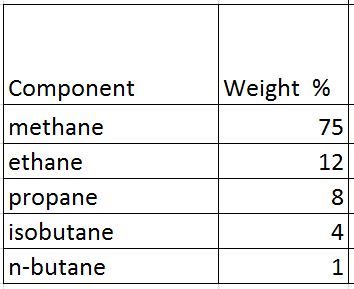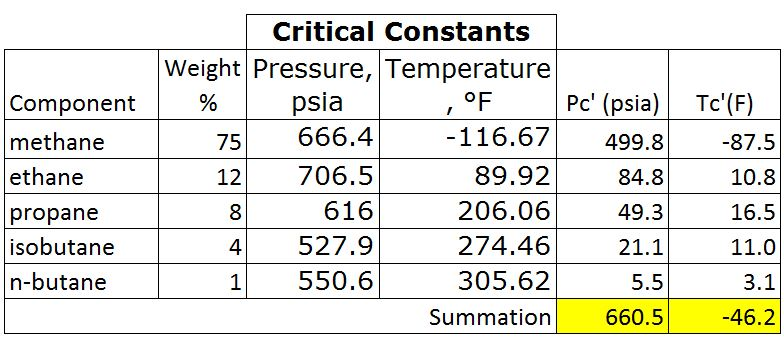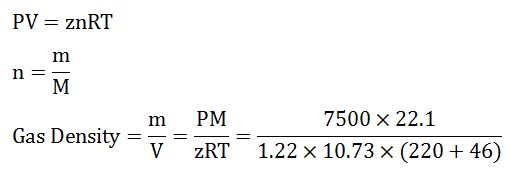# Example of Real Gas Calculation

This example will demonstrate how to calculate the compressibility of real gas in order to determine gas density and specific gravity at a specific condition.

Calculate the following based on the given condition:

1) Density of this gas under the reservoir conditions of 7,500psia and 220ºF,

2) Specific gravity of the gas.

Gas component is shown in Table 1Table 1 – Gas Component

Average density of air = 28.96 lb/cu-ft

## Solution

• Determine critical pressure and temperature of gas mixtures using Kay’s ruleTable 2 – Critical Pressure and Temperature

Note: critical pressure and temperature can be found from this link – https://www.drillingformulas.com/determine-compressibility-of-gases/

Pc’ = Σyipci = 660.5 psia

Tc’ = ΣyiTci = -46.2 F = -46.2 +460 F = 413.8 RTable 3 – Pc’ and Tr’ by Kay’s Rule

• Calculate Tr and Pr

Tr = T ÷Tc

Tr = (220+460) ÷ (-46.2+460)

Tr = 1.64

Note: temperature must be in Rankin.

Rankin = Fahrenheit + 460

For the critical temperature calculation, it can be converted the critical temperature from F to R before calculating Tc’. This will still give the same result.

Pr = P ÷ Pc

Pr = 7500 ÷ 660.5 = 11.4

• Read the compressibility factor (z) from the chart.

z = 1.22Figure 1-z-factor from the Standing and Katz Chart

• Calculate average molar mass

Average Molar Mass = Σyi×Mi = 22.1 lbTable 4 – Average Molar Mass of Gas

• Calculate density of gas from the equation below;Gas Density = 18.6 lb/cu-ft

• Calculate gas specific gravity from the equation below;

SG = Gas Density ÷ Air Density

SG = 18.6 ÷ 28.96

SG = 0.64

Summary:

Gas Density = 18.6 lb/cu-ft

SG = 0.64

We wish that this example will help you understand to determine z-factor and use it to calculate any related information.

References

Abhijit Y. Dandekar, 2013. Petroleum Reservoir Rock and Fluid Properties, Second Edition. 2 Edition. CRC Press.

L.P. Dake, 1983. Fundamentals of Reservoir Engineering, Volume 8 (Developments in Petroleum Science). New impression Edition. Elsevier Science.

Tarek Ahmed PhD PE, 2011. Advanced Reservoir Management and Engineering, Second Edition. 2 Edition. Gulf Professional Publishing.

Share the joy
Tagged , , , . Bookmark the permalink.